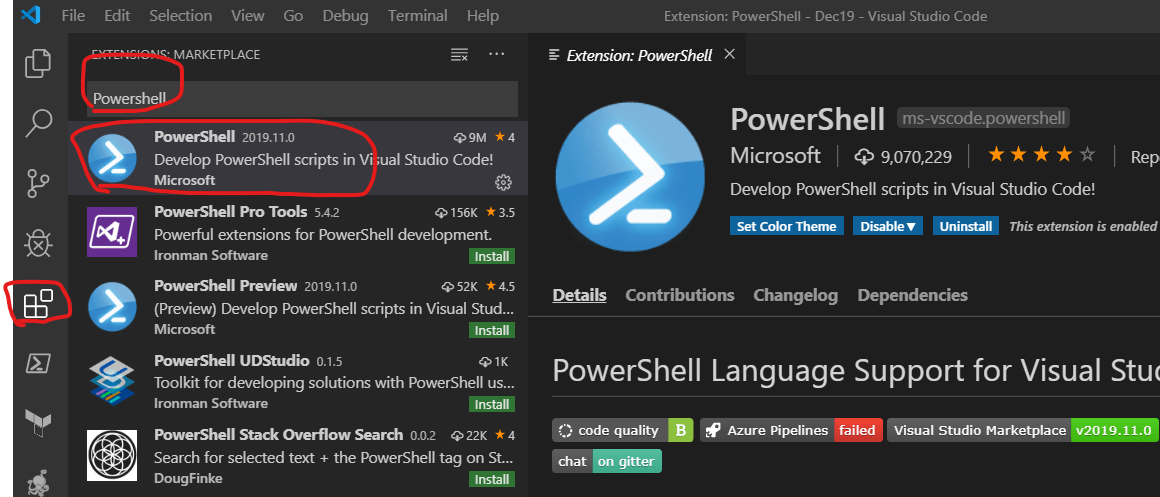## Understanding Powershell cmd-lets

• Cmdlets will always return objects.
• Every object will have
• Methods
• Properties
• Cmdlets are available by default in Powershell. Additional Cmdlets can be installed in the system using modules from Powershell Gallery.

## Writing Scripts in Powershell

• Create a file with extension PS1
• To Start Scripting we need
• DataTypes
• Variables
• Operators
• Preparation for Powershell Scripting
• Powershell ISE
• Visual Studio Code
• Visual Studio Code Preparation:
• Install VS Code
• Install Powershell Extension### Writing a Prime number is Powershell

``````\$number = 99

\$index = 2

\$is_prime = \$true
while (\$index -lt \$number) {
if (\$number % \$index -eq 0) {
\$is_prime = \$false
break
}

\$index += 1

}
if (\$is_prime -eq \$true) {
echo "Prime"
}
else {
echo "Not Prime"
}
``````

• Reads the input from user and type is always string
• If you need other types, do the conversions.
• For example refer code below
``````\$enterednumber = Read-Host -Prompt 'Enter number'
\$type = \$enterednumber.GetType().FullName
echo "Number entered is \$enterednumber and type is \$type "
\$number = [convert]::ToInt32(\$enterednumber)
\$type = \$number.GetType().FullName
echo "Number entered is \$number and type is \$type "

\$index = 2

\$is_prime = \$true
while (\$index -lt \$number) {
if (\$number % \$index -eq 0) {
\$is_prime = \$false
break
}

\$index += 1

}
if (\$is_prime -eq \$true) {
echo "Prime"
}
else {
echo "Not Prime"
}
``````
1. param:
• Define parameters in PS1 file by using param block
``````param (
[int32] \$a,
[int32] \$b
)
\$result = \$a*\$b
echo "\$a * \$b = \$result"
``````
``````* Calc.ps1 add/mul <numbers>
``````
``````param(
[Parameter(Mandatory=\$true)]
[string] \$operation,
[Parameter(Mandatory=\$true)]
[double] \$num1,
[Parameter(Mandatory=\$true)]
[double] \$num2
)
\$result = \$num1+\$num2
echo "\$num1 + \$num2 = \$result"
}
elseif (\$operation -eq 'mul') {
\$result = \$num1*\$num2
echo "\$num1 * \$num2 = \$result"

}
else {
echo "\$operation is not supported"
}
``````

## Environment Variables

• Environmental Variables in Powershell are much like other variables, but they have different syntax
``````\$env:<variablename>
``````
• To Get Environmental varaible
``````echo \$env:Path
``````
• To Set a new Environment variable
``````\$env:test = "C:\"
``````

This site uses Akismet to reduce spam. Learn how your comment data is processed.